﻿ CalcuDoku решение
 ВходРегистрация# CalcuDoku решение

CalcuDoku are math and logic puzzles which require placing numbers in a grid according to the rules. Each puzzle consists of a grid containing blocks surrounded by bold lines. The object is to fill all empty squares so that the numbers 1 to N (where N is the number of rows or columns in the grid) appear exactly once in each row and column and the numbers in each block produce the result of the operation shown in the top-left corner of the block. In CalcuDoku a number may be used more than once in the same block.

CalcuDoku puzzles are available in different variants. SingleOp CalcuDoku uses one math operation which is either addition or multiplication. Similarly, DualOp CalcuDoku uses addition and subtraction or multiplication and division while QuadOp CalcuDoku uses addition, subtraction, multiplication and division. The math operation used in each block is shown in its top-left corner except for SingleOp CalcuDoku where all blocks use one math operation as shown above the grid.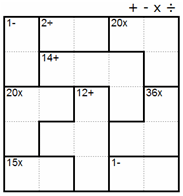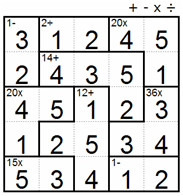## Starting with the givens

Some CalcuDoku puzzles, especially easy ones, contain blocks consisting of a single square. These are actually given clues and the number to be placed is simply the number in the top-left corner of the single square block regardless of which math operations are shown above the grid. We can therefore place 2 in the example below.## Unique block techniques

Similar to Kakuro and Sum Sudoku, CalcuDoku puzzles often have situations where only a single combination of numbers can fit in a block. These situations are useful when starting to solve a puzzle especially if they appear in straight blocks. Here are some ways of using the unique block techniques:

#### Unique block technique 1:

In the left column of the SingleOp CalcuDoku puzzle below we see a block of two squares with the sum 4. Since CalcuDoku rules don’t allow the same number to appear more than once in a row or a column the only combination to satisfy this requirement is 1+3 though we don’t yet know in which order the numbers are placed. We can therefore place 4 in the bottom square of the left column, and 3, the block remainder, in the adjacent square.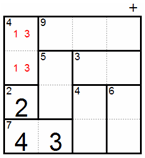#### Unique block technique 2:

We can use the same technique for blocks with multiplication. In the bottom row of the example below is a block of two squares with the multiplication product 2. This means the only combination can be 1x2 though we don’t yet know in which order the numbers are placed. Since we also have 4 in the bottom row, the only candidate for the square on the right side of the bottom row is 3. After placing 3 we can also place 1, the block remainder, in the square above it.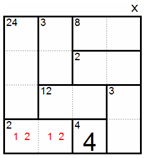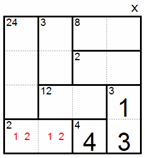#### Unique block technique 3:

In the bottom row of the example below we see a two-square block with the sum 4. Since CalcuDoku rules don’t allow the same number to appear more than once in a row or a column the only combination can be 1+3. However, the top-left square already contains 3 which means there is only one way to place 1 and 3 in the bottom row as shown in the diagram on the right.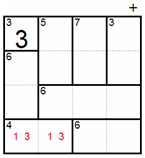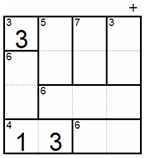#### Unique block technique 4:

This example is similar to the ones above except there are two empty squares at the top of the left column and the candidates for those squares are 4 and 5. However, 5 is too large to place in the top-left block so 4 remains the only candidate as shown in the right diagram below. We can now place 1, the block remainder, in the adjacent square.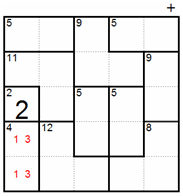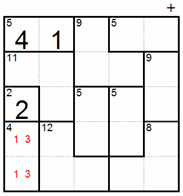#### Unique block technique 5:

We can use the same technique for blocks with multiplication. The top row of the example below contains a three-square block with the multiplication product 6. Since the only combination allowed is 1x2x3 we can deduct that 4 and 5 are in the remaining two squares in the top row. However, we can’t place 5 in the right-most square because 8 can only be the result of 2x4. Therefore we must place 4 in the top-right square and 2, the block remainder, in the square under it.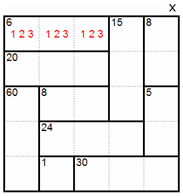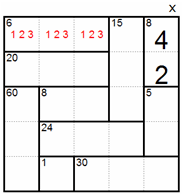#### Unique block technique 6:

Below is a DualOp CalcuDoku puzzle which uses multiplication and division operations. Similar to the previous examples, the only candidate for the left square in the second row of the puzzle below is 3. After placing 3 we can also place 1 in the square below since it is the only way to get the division result of 3.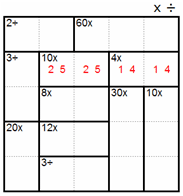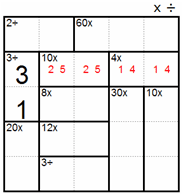#### Unique block technique 7:

Here is another example of using the unique block technique in a DualOp CalcuDoku puzzle with multiplication and division. Let’s examine the block with 2÷ in the left column. There are only two combinations possible, 2÷1 and 4÷2 which make 1, 2 and 4 the only possible candidates for this block. However, the fourth row already contains 1 and 4 leaving 2 as the only possibility for the left square in the fourth row. Unlike previous examples, we cannot yet place the block remainder since both 1 and 4 are possible.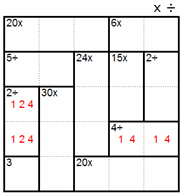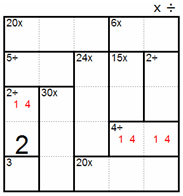#### Unique block technique 8:

Sometimes it is harder to identify unique block techniques as shown in the example below. The only combination possible for the top block in the right column is 1+2. However, when we examine the three-square block in the first row we see only 2+4+5 is possible which excludes 2 from the top square in the right column and leaves 1 as its only candidate. After placing 1 in the top square of the right column we can also place 2, the block remainder, in the square underneath.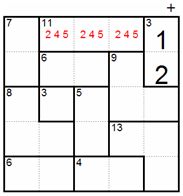## Single candidate techniques

According to the first rule of CalcuDoku a number can appear only once in the same row or column which means we can sometimes use Sudoku solving techniques as well. Let’s look at the square with the question mark in the partially solved puzzle below. Since 1 and 5 have already been placed in other squares in this row, and since 2 and 4 have already been placed in other squares in this column, the only candidate left is 3.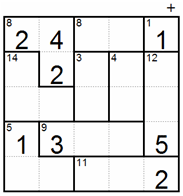## Hidden single techniques

Sometimes there are situations where a number is placed in a square simply because it cannot be placed in any other square of that row or column. This process of elimination is named hidden single, same way as it is named in Sudoku.

#### Hidden single technique 1:

Let’s look at the left column of the QuadOp CalcuDoku puzzle below. In the bottom block the only combination can be 4÷1, though we don’t yet know in which order the numbers will be placed. Similarly, the only combination in the center block can be 5-2. Although 4-1 also gives the correct result for the center block operation, 1 and 4 are already used in the left column from the previous step. Now, if we put everything together we see 3 cannot be placed in either of these blocks which means it can only be placed in the top square of the left column.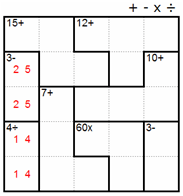#### Hidden single technique 2:

A similar situation occurs in the third row of the partially solved puzzle below, where 5 can only be placed in the second square from the right.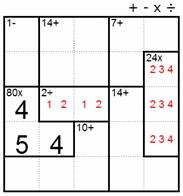## Grid remainder techniques

Grid remainder, a handy technique in many situations, uses a different approach to analyzing and solving CalcuDoku puzzles. Grid remainder is based on the fact that the sum (or product) of all squares in a row or column of a CalcuDoku puzzle of a given size is always the same. For example, in a 4x4 puzzle the sum of all squares in any row or column is always 1+2+3+4=10. Similarly, the product of all squares in any row or column is always 1x2x3x4=24. Here are a few examples for the grid remainder technique:

#### Grid remainder technique 1:

The top row of the puzzle below has two fully contained blocks shaded in gray, one with the sum 2 (which is actually a given) and the other with the sum 8. Together these two blocks sum up to 2+8=10, but we know that any row or any column in a 5x5 CalcuDoku puzzle must sum up to 1+2+3+4+5=15. This means the difference 15-10=5 is caused by the un-shaded square in the top row and we can therefore place in it 5.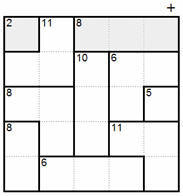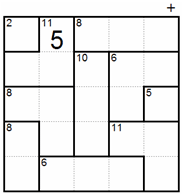#### Grid remainder technique 2:

Here is another example with a twist. The left column contains two blocks shaded in gray, one with the sum 10 which is fully contained in the left column, and one with the sum 9 which has only two squares contained in the left column. Together these two blocks sum up to 10+9=19, but we know that any row or any column in a 5x5 CalcuDoku puzzle must sum up to 1+2+3+4+5=15. This means the difference 19-15=4 is caused by the square in the bottom block which isn’t contained in the left column and we can therefore place in it 4.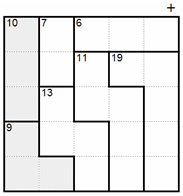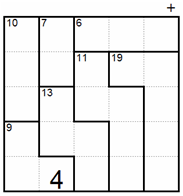#### Grid remainder technique 3:

The grid remainder technique is also useful for rows and columns containing blocks with multiplication. The right column of the puzzle below contains two blocks shaded in gray, one with the product 8 which is fully contained in the right column, and one with the product 60 which has only two squares contained in the right column. Together these two blocks have a product of 8x60=480, but we know that the product of any row or any column in a 5x5 CalcuDoku puzzle must be 1x2x3x4x5=120. This means the quotient 480÷120=4 is caused by the square which isn’t contained in the right column and we can therefore place in it 4.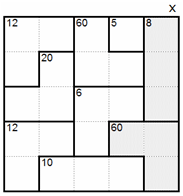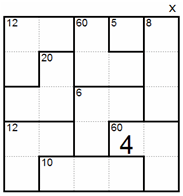## Intra block techniques

Intra block techniques cover a range of methods which help find both the value and the location in which numbers should be placed in a block. Here are a few examples of how to use intra block techniques:

#### Intra block technique 1:

In the example below, the only combination for the sum 14 in the shaded block is 4+5+5. This situation is true for all three-square L-shaped blocks of a 5x5 puzzle. In addition to knowing which numbers should be placed we also know where they should be since the 5’s must be diagonal to avoid a number appearing in the same row or column more than once.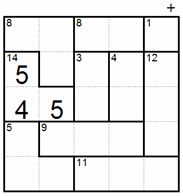#### Intra block technique 2:

Here is another example where the intra block technique can help find some of the numbers in a block. In the puzzle below three out of the four squares of the shaded block are contained in one column. This means the only possible combination for these three squares is 1+2+3=6 because any other combination will reach or exceed 7, the sum of this block, thus not allowing any number in its fourth square. We can therefore place 1, the block remainder calculated by 7-6, in the fourth square.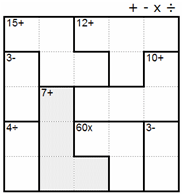#### Intra block technique 3:

Intra block techniques can be used equally well for blocks with multiplication. In the example below, the only combination for the product 80 in the shaded block is 4x4x5. This situation is true for all three-square L-shaped blocks of a 5x5 puzzle. In addition to knowing which numbers should be placed we also know where they should be since the 4’s must be diagonal to avoid a number appearing in the same row or column more than once.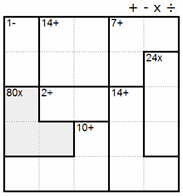#### Intra block technique 4:

Larger blocks present more challenging methods of using the intra block technique. Let’s look at the shaded block with the sum 8 in the partially solved puzzle below. Using the minimum sum approach, the smallest possible sum in the top row of this block is 1+2=3 and the smallest possible sum in the second row is also 1+2=3. This means that 3+3=6 out of the sum 8 in this block is already accounted for leaving only 1 and 2 as candidates for the last square. However, since 2 is already used in the same column, 1 must be placed in the bottom square of this block.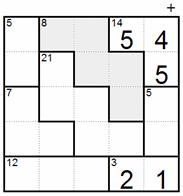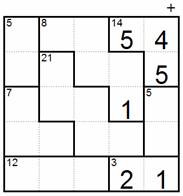#### Intra block technique 5:

In 5x5 CalcuDoku puzzles, the only combinations for the product 32 in four squares are1x2x4x4 and 2x2x2x4. If we look at the shaded L-shaped block below it is obvious that the second combination is not possible because no matter how we place the numbers there will always be 2 in the same row or column. This means only 1x2x4x4 is allowed and 4 must be placed in the left square to avoid conflict with the other 4.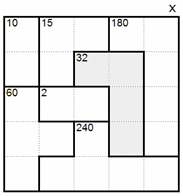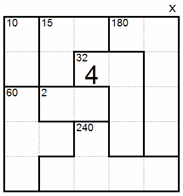## Advanced techniques

The techniques described so far won’t be enough to solve hard puzzles. For this you will need advanced techniques to work out many special and interesting logic situations. Most advanced techniques use recursion, a looking ahead process of making an assumption and checking for conflicts one or two steps ahead. Although the results of advanced techniques may sometimes be similar to those of the previous techniques, there is a big difference because solvers must look ahead to find the logical conflict or contradiction. Here are some examples of advanced techniques to solve special situations. You will develop many more of your own when you start solving hard CalcuDoku puzzles by yourself:

#### Advanced technique 1:

Below is a partially solved CalcuDoku 5x5 puzzle. We know the left column must contain 5 so let’s assume it is placed in one of the red dotted squares. This means the minimum possible sum of the shaded block will be 1+2+5=8 in the left column, 1+2+3=6 in the second column and 1+2=3 in the third column, which altogether add up to 8+6+3=17. Since 17 is more than the sum of this block, we know our initial assumption is incorrect and 5 must be placed in the bottom row of the left column.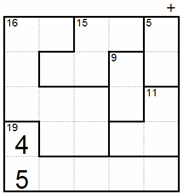#### Advanced technique 2:

This technique is similar to the one above except it uses an opposite assumption to prove a number must be used in a certain area. Below is a partially solved CalcuDoku 5x5 puzzle. Let’s assume 5 in the second row is not placed in one of the red dotted squares. This means the maximum possible sum of the shaded block will be 2+5=7 in the top row, 2+3+4=9 in the second row and 5 in the third row, which altogether add up to 7+9+5=21. Since 21 is less than the sum of this block, we know our initial assumption is incorrect and 5 must be placed in one of the squares with the red dots. Although we don’t know in which red dotted square 5 should be placed, we now know exactly where 1 and 5 are placed in the left column.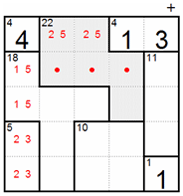#### Advanced technique 3:

As with any 5x5 CalcuDoku puzzle, the sum of all squares in the left column of the shaded block below must be 1+2+3+4+5=15. Since the total sum of this block is 20, we still need to place numbers in the two red dotted squares which together sum up to 5. Now let’s examine the second column from the left to see where 5 can be placed. We can’t place 5 in a red dotted square because we will exceed the sum 20 in the shaded block. We also can’t place 5 in the L-shaped block above the red dotted squares because only 1x1x4 or 1x2x2 can produce 4. Therefore 5 can only be placed in the top square of the second column as shown in the right diagram below.